# LOG#071. Pavšič Units.

Following the thread I began in the previous blog post, today we are going to learn about a non common but very general and universal system of natural units. They were officiallly introduced without name in the literature, until a physicist realized its importance, to my knowledge, by first time.

In his book, The Landscape of Theoretical Physics: A Global View, available here and here for free,  the slovenian physicist Matej Pavšič introduced a dilationally invariant system of units, where “all” the fundamental “constants” are set to the unit. More precisely, he defines the D-system or  Pavšič units M system characterized by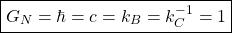and where the Coulomb constant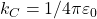so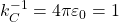. This sistem of units, let me call it M (for Matej) or D-system (we will see later the meaning of D), is very useful but it has not been discussed in the literature deeper, unlike the case of natural HEP units, Stoney units or the Planck units.

We have seen in the previous post that addapted system of units do exist where the physical quantities lack any spurious conversion constant (like c or any power or it, the Planck constant and any power of it, and so on). Indeed, we could say that these “fundamental” constants are yet there, but they are “invisible” due to the election of some clever system of units ( the so-called natural units).

The M system of units or D-system ( following M. Pavšič) defines a dilationally invariant system of units, and it is the fact that this system has some special behaviour under “dilatations” why it receives such a name. In the MKSA system, the quantities of this system are written as follows: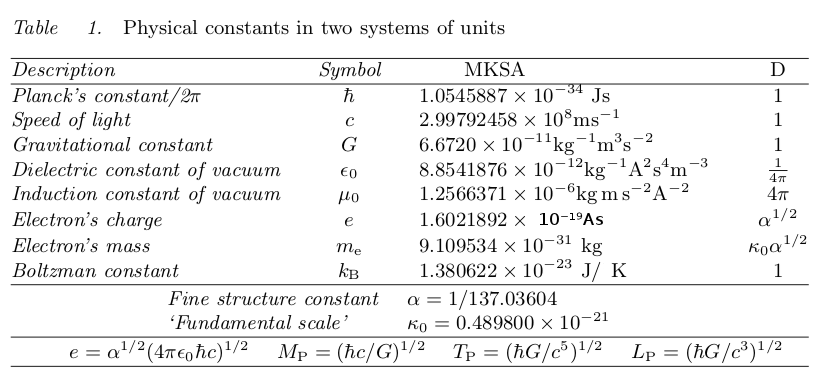We can compare MKSA units and D-system units. Firstly, in MKSA, we have: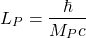However, in D units, we also have: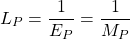Of course, this result is the same than the Planck system of units. In addition to these equations, we can also define: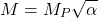and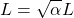Furthermore, we can also set the electric charge to “mass” as follows (in the D-system):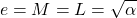Length is defined as the radius a classical particle with mass M and a charge elementary e would have. In MKSA, we easily derive: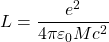By the other hand, in terms of units D, it yields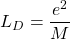Thus, length seems to be the ratio between “squared charge” and “mass” somehow from D units, i.e., if we further impose that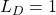, we would “deduce” that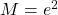or more generally the relationship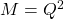(recall the striking convergence to the charge-mass equivalence from these dimensional arguments as it does also happen in black hole physics!). The classical radius of the electron is, as every physicist knows: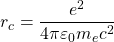in MKSA units, but it isin D units. Taking the ratio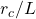, it yields:and thus, we can write in D units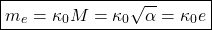This equation says that mass is equivalent to charge up to a tiny constant, denoted by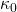. This dimensionless constant is very mysterious and it has not been discussed in the literature as far as I know excepting in that M. Pavšič book! Its reciprocal value is also a curious number:This number is roughly 1/295 times the Avogrado constant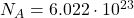. Matej  Pavšič also mentions that the ratioor its reciprocal represents the “scale of length” of the classical electron radius relative to the length L, and we have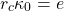The next table provides the conversion factors between D-system units and MKSA units: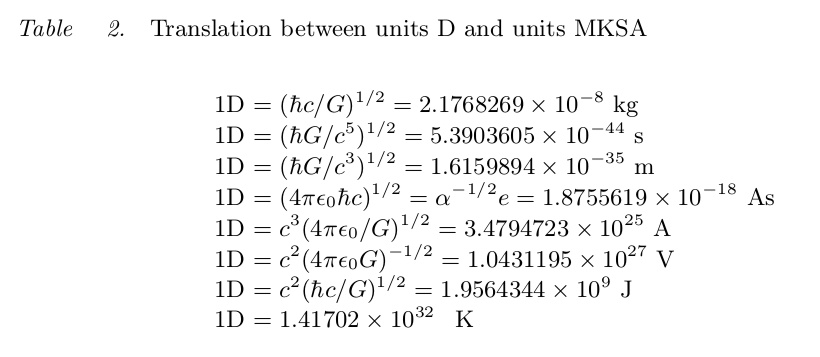Furthermore, the D unitsand also, plus the conventional and commonly known asare also invariant under dilatations! It is quite a claim, so let me review the arguments that  Pavšič gives in his book. If we make a dilatation transformation of position, linear momentum, mass, force and acceleration (after a  “scale transformation”), we write: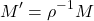Instead of the inhomogeneous coordinates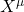one can introduce the homogeneous coordinateswhereare invariant under dilatations provided that the quantity κ transforms as follows:For instance, if initially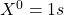, then after applying a dilatation, say by the factor ρ = 3, we have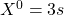. Setting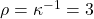fixes the scale transformation relative to some homogeneous coordinate.

If we write a given equation we can check its consistency by comparing the dimension of its left hand and right hand side. In the MKSA system the dimensional control is in checking the powers of meters, kilograms, seconds and ampères on both sides of the equation. Moreover, even the Einstein Field Equations of gravity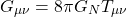can be expressed in units where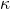is “visible” as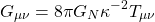If we choose κ = 1 then the equations for this particular choice correspond to the usual Einstein Field  Equations, and we may use either the MKSA system or the D system.

In the D-system, some equations simplify further, even more than in Planck units! Let’s see some interesting uncommon equations in this new system of units:The final part of this nice post corresponds to a nice exercise included in the  Pavšič book. Suppose you are given an equation in the D system, e.g.,How can we turn it to usual MKSA units? Using conversion constants, we can rewrite the previous equation in the next way: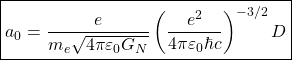If we put 1D = 1 then the right hand side of the latter equation is a pure dimensionless quantity. If we wish to obtain a quantity of the dimension of length we have to insert the factor:and we finally obtain the Böhr radius: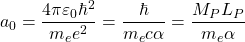Instead of rewriting equations from the D system in the MKSA system, we can keep all the equations in the D system and perform all the algebraic and numerical calculations in the D units. If we wish to know the numerical results in terms of the MKSA units, we can use the numbers of Table 2 above. For instance, in the previous example, going backwards, we haveTaking D units of length, from Table 1, we read off that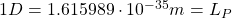and we obtain the classical result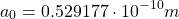Let me quote M. Pavšič:

“(…)However, I do not propose to replace the international MKSA system with the D system. I only wish to recall that most modern theoretical works do not use the MKSA system and that it is often very tedious to obtain the results in meters, seconds, kilograms and ampères. (…)besides the Planck length, Planck time and the Planck mass, which are composed of the fundamental constants,, we have also introduced (see Table 2) the corresponding electromagnetic quantity, namely the charge(or, equivalently, the current and the potential difference), by bringing into play the fundamental constant. We have then extended the Planck system of units in which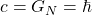to a more complete system wherein order to incorporate all known sorts of physical quantities(…).”

Remarkably, at the end, M. Pavšič points out a classical paper by J.M. Levy-Leblond, see here:http://inspirehep.net/record/128108 , in which, Matej says, is clearly stated that our progress in understanding the unity of nature follows the direction of eliminating from theories various (inessential) numerical constants with the improper name of “fundamental” constants. In fact, those constants are merely the constants which result from our unnatural choice of units, the choice due to our incomplete understanding of the unified theory behind.

Matej Pavšič has become a blogger recently (you can find his site in my links on the right or here) and he has also a nice webpage about his research and lots of interesting topics. He has also studied tachyons, extended objects, geometric algebra, Clifford spaces (C-spaces), brane worlds, relativistic dynamics with an invariant evolution parameter (the so-called Stuckelberg formalism), Kaluza-Klein theories and many other interesting topics. With Carlos Castro Perelman, he is one of the proponents of the theory of relativity in Clifford spaces (C-spaces), an extension of special relativity/general relativity/QFT including grassmann valued quantities and arbitrary signatures on equal footing, but living NOT in spacetime but in some kind of nontrivial and purely algebraic space. You can visit his page and have a look to his works here.

Have you liked this post? Let me know. And be right back for my next communication! Physics is cool, Physmatics is cool too and Mathematics is an incredible language for Physmatics!

1. Thanks for reviewing the units discussed in Appendix A of my book “The Landscape of Theoretical Physics: A Global View; From Point Particles to the Brane World and Beyond, in Search of a Unifying Principle” (Kluwer Academic, 2001). In fact, these are just Planck units, the only difference is in putting, besides.
• You are welcome, Matej! Well, you are right BUT you have the credit to have realized for the first time to my knowledge that you can fix to the unit the remaining constant, the Coulomb’s constant, or equivalently its reciprocal, i.e., dimensionally, a electrical permitivity/condenser or capacitor over length. Interestingly, I calculated the Planck’s capacitor value in my previous post (it is essentially the Planck length over). The “vacuum capacitor” is related somehow to the Planck’s length through. It is striking that from a purely classical and dimensional arguments we can “derive” that vacuum is not only “elastic”/gravitational like a deformable medium (Sakharov’s induced gravity comes to my mind when I look at) but also “electric”. And moreover, I am yet trying to understand what kind of “Physics” is behind. PS: Keep pushing with your blog! It will be great too!